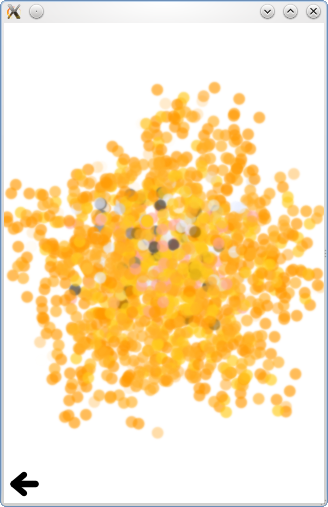# Qt Quick Particles Examples - CustomParticle

This is a collection of examples using CustomParticle in the QML particle system.This is a collection of small QML examples relating to using CustomParticle in the particle system. Each example is a small QML file emphasizing a different way to use CustomParticle.

Blur Particles adds a blur effect to the particles, which increases over the particle's life time. It uses a custom vertex shader:

```vertexShader:"
uniform lowp float qt_Opacity;
varying lowp float fBlur;

void main() {
defaultMain();
highp float t = (qt_Timestamp - qt_ParticleData.x) / qt_ParticleData.y;
highp float fadeIn = min(t * 10., 1.);
highp float fadeOut = 1. - max(0., min((t - 0.75) * 4., 1.));

fBlur = max(0.2 * t, t * qt_ParticleR);
}
"```

to propagate life time simulation to a custom fragment shader:

```fragmentShader: "
uniform sampler2D source;
uniform sampler2D blurred;
varying highp vec2 qt_TexCoord0;
varying highp float fBlur;
void main() {
gl_FragColor = mix(texture2D(source, qt_TexCoord0), texture2D(blurred, qt_TexCoord0), min(1.0,fBlur*3.0)) * fFade;
}"```

which has access to both the normal image sampler and a blurred sampler, the image plus a ShaderEffect.

Fragment Shader just uses the particle system as a vertex delivery system.

```fragmentShader: "
varying highp vec2 fPos;
varying highp vec2 qt_TexCoord0;
void main() {//*2 because this generates dark colors mostly
highp vec2 circlePos = qt_TexCoord0*2.0 - vec2(1.0,1.0);
highp float dist = length(circlePos);
highp float circleFactor = max(min(1.0 - dist, 1.0), 0.0);
gl_FragColor = vec4(fPos.x*2.0 - fPos.y, fPos.y*2.0 - fPos.x, fPos.x*fPos.y*2.0, 0.0) * circleFactor * fFade;
}"```

Image Colors uses CustomParticle to assign colors to particles based on their location in a picture. The vertex shader,

```vertexShader:"
uniform highp float maxWidth;
uniform highp float maxHeight;
varying highp vec2 fTex2;
uniform lowp float qt_Opacity;

void main() {

fTex2 = vec2(qt_ParticlePos.x, qt_ParticlePos.y);
//Uncomment this next line for each particle to use full texture, instead of the solid color at the center of the particle.
//fTex2 = fTex2 + ((- qt_ParticleData.z / 2. + qt_ParticleData.z) * qt_ParticleTex); //Adjusts size so it's like a chunk of image.
fTex2 = fTex2 / vec2(maxWidth, maxHeight);
highp float t = (qt_Timestamp - qt_ParticleData.x) / qt_ParticleData.y;
fFade = min(t*4., (1.-t*t)*.75) * qt_Opacity;
defaultMain();
}
"```

passes along the starting position for each vertex to the fragment shader,

```fragmentShader: "
uniform sampler2D particleTexture;
uniform sampler2D pictureTexture;
varying highp vec2 qt_TexCoord0;
varying highp vec2 fTex2;